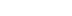/

# Sponsored Feature: Rasterization on Larrabee -- Adaptive Rasterization Helps Boost Efficiency

In a detailed Intel-sponsored technical article, part of its Visual Computing section on Gamasutra, veteran game programmer and architect Mike Abrash (Quake) goes in-depth on how Intel's upcoming Larrabee architecture pipeline deals intelligently with rasterization.

[In a detailed Intel-sponsored technical article, part of its Visual Computing section on Gamasutra, veteran game programmer and architect Mike Abrash (Quake) goes in-depth on how Intel's upcoming Larrabee architecture pipeline deals intelligently with rasterization.]

You don't tug on Superman's cape

You don't spit into the wind

You don't pull the mask off that old Lone Ranger

And you don't mess around with Jim

The first time you heard the chorus of "You Don't Mess Around with Jim," you no doubt immediately wondered why Jim Croce left out "And you don't rasterize in software if you're trying to do GPU-class graphics, even on a high-performance part such as Larrabee." That's just common sense, right? It was obvious to me, anyway, that Larrabee was going to need a hardware rasterizer. But maybe Jim knew something I didn't, because it turned out I was wrong, and therein lies a tale.

First, though, let's back up a step and get some context.

### Parallel programming for semi-parallel tasks

In Look at the Larrabee New Instructions (LRBni), I presented an overview of Larrabee, an upcoming multithreaded, vectorized, many core processor from Intel. It may not be entirely apparent from that article, but the LRBni contains everything you need to do a great job running code that's designed to be vectorized, such as HLSL shaders. But what about tasks that aren't inherently scalar (in particular, where the iterations aren't serially dependent), and that at the same time can't be efficiently parallelized in any obvious way, and for which the usual algorithms aren't easily vectorized?

In the process of implementing the standard graphics pipeline for Larrabee, we (the Larrabee team at Intel's partner RAD Game Tools - Atman Binstock, Tom Forsyth, Mike Sartain, and me) have gotten considerable insight into that question, because while the pipeline is largely vectorizable, there are a few areas in which the conventional approaches are scalar, and where some degree of serialization is unavoidable.

This article takes a close look at how we applied the Larrabee New Instructions to one of those problem areas - rasterization - in the process, redesigning our implementation so it was far more vectorizable so it took advantage of the strengths of Larrabee's CPU-based architecture. Needless to say, performance with the Larrabee New Instructions will vary greatly from one application to the next - there's not much to be done with purely scalar code - but diving into Larrabee rasterization in detail gives you a good sense of what it's like to apply the Larrabee New Instructions to at least one sort of non-obvious application.

To avoid potential confusion, let me define "rasterization": For our present purposes, it's the process of determining which pixels are inside a triangle, and nothing more. In this article, I won't discuss other aspects of the rendering pipeline, such as depth, stencil, shading, or blending, in any detail.

### Rasterization Was the Problem Child - But Less Problematic Than We Thought

When we look at applying the Larrabee New Instructions to the graphics pipeline, for the most part, it's easy to find 16-wide work for the vector unit to do. Depth, stencil, pixel shading, and blending all fall out of processing 4×4 blocks, with writemasking at the edges of triangles. Vertex shading is not quite a perfect fit, but 16 vertices can be shaded and cached in parallel, which works well because vertex usage tends to be localized. Setting up 16 triangles at a time also works pretty well; there's some efficiency loss due to culling, but it still yields more than half of maximum parallelism.

That leaves rasterization, which is definitely not easy to vectorize with enough performance to be competitive with hardware. As an aside, the Larrabee rasterizer is not, of course, the only rasterizer that could be written for Larrabee. There are other rasterizers for points and lines, and of course, there may be other future rasterizers for interesting new primitive types. In fact, I suggest you write your own - it's an interesting problem! But as it doesn't have a more formal name, I'll just refer to the current general-purpose triangle rasterizer as "the Larrabee rasterizer" in this article.

The Larrabee rasterizer was at least the fifth major rasterizer I've written, but while the others were loosely related, the Larrabee rasterizer belongs to a whole different branch of the family - so different, in fact, that it almost didn't get written. Why? Because, as is so often the case, I wanted to stick with the mental model I already understood and was comfortable with, rather than reexamining my assumptions. Long-time readers will recall my fondness for the phrase "Assume nothing"; unfortunately, I assumed quite a lot in this case, proving once again that it's easier to dispense wise philosophy than to put it into action!

Coming up with better solutions is all about trying out different mental models to see what you might be missing, a point perfectly illustrated by my efforts about 10 years ago to speed up a texture mapper. I had thrown a lot of tricks at it and had made it a lot faster, and figured I was done. But just to make sure, I ran it by my friend David Stafford, an excellent optimizer. David said he'd think about it and would let me know if he came up with anything.

When I got home that evening, there was a message on the answering machine from David, saying that he had gotten two cycles out of the inner loop. (It's been a long time, and my memories have faded, so it may not have been exactly two cycles, but you get the idea.) I called him back, but he wasn't home (this was before cellphones were widespread). So all that evening I tried to figure out how David could have gotten those two cycles. As I ate dinner, I wondered; as I brushed my teeth, I wondered; and as I lay there in bed not sleeping, I wondered. Finally, I had an idea - but it only saved one cycle, not two. And no matter how much longer I racked my brain, I couldn't get that second cycle.

The next morning, I called David, admitted that I couldn't match his solution, and asked what it was. To which he replied: "Oh, sorry, it turned out my code doesn't work."

It's certainly a funny story, but more to the point, the only thing keeping my code from getting faster had been my conviction that there were no better solutions - that my current mental model was correct and complete. After all, the only thing that changed between the original solution and the better one was that I acquired the belief that there was a better solution - that is, that there was a better model than my current one. As soon as I had to throw away my original model, I had a breakthrough.

Software rasterization is a lot like that.

The previous experience that Tom Forsyth and I had with software rasterization was that it was much, much slower than hardware. Furthermore, there was no way we could think of to add new instructions to speed up the familiar software rasterization approaches, and no obvious way to vectorize the process, so we concluded that a hardware rasterizer would be required. However, this turned out to a fine example of the importance of reexamining assumptions.

In the early days of the project, as the vector width and the core architecture were constantly changing, one of the hardware architects, Eric Sprangle, kept asking us whether it might be possible to rasterize efficiently in software using vector processing. We kept patiently explaining that it wasn't. Then, one day, he said: "So now that we're using the P54C core, do you think software rasterization might work?" To be honest, we thought this was a pretty dumb question because the core didn't have any significant impact on vector performance; in fact, we were finally irritated enough to sit down to prove in detail - with code - that it wouldn't work.

And we immediately failed. As soon as we had to think hard about how the inner loop could be structured with vector instructions, and wrote down the numbers - that is, as soon as our mental model was forced to deal with reality - it became clear that software rasterization was in fact within the performance ballpark. After that, it was just engineering, much of which we'll see shortly.

First, though, it's worth pointing out that, in general, dedicated hardware will be able to perform any specific task more efficiently than software; this is true by definition because dedicated hardware requires no multifunction capability, so in the limit, it can be like a general-purpose core with the extraneous parts removed. However, by the same token, hardware lacks the flexibility of CPUs, and that flexibility can allow CPUs to close some or all of the performance gap. Hardware needs worst-case capacity for each component, so it often sits at least partly idle; CPUs, on the other hand, can just switch to doing something different, so ALUs are never idle. CPUs can also implement flexible, adaptive approaches, and that can make a big difference, as we'll see shortly.

### Why Rasterization Was the Problem Child

I can't do a proper tutorial on rasterization here, so I'll just run through a brief refresher. For our purposes, all rasterization will be of triangles. There are three edges per triangle, each defined by an equation Bx+Cy relative to any point on the edge, with the sign indicating whether a point is inside or outside the edge. Both x and y are in 15.8 fixed-point format, with a range of [-16K, +16K). The edge equations are tested at pixel or sample centers, and for cases where a pixel or sample center is right on an edge, well-defined fill rules must be observed (in this case, top-left fill rules, which are generally implemented by subtracting 1 from the edge equation for left edges and flat-top edges). Rasterization is performed with discrete math, and must be exact, so there must be enough bits to represent the edge equation completely. Finally, multisampled antialising must be supported.

Let's look at a quick example of applying the edge equation. Figure 1 shows an edge from (12, 8) to (4, 24). The B coefficient of the edge equation is simply the edge's y length: (y1 - y0). The C coefficient is the negation of the edge's x length: (x0 - x1). Thus, the edge equation in Figure 1 is (24 - 8)x + (12 - 4 )y. Since we only care about the sign of the result (which indicates inside or outside), not the magnitude, this can be simplified to 2x + 1y, where the x value used in the equation is the distance between the point of interest and any point at which the equation is known to be zero (which is to say, any point on the line). Usually, a vertex is used; for example, as the vertex at (12, 8) is used in Figure 1. All points on the edge have the value 0, as can be seen in Figure 1 for the point on the line at (8, 16).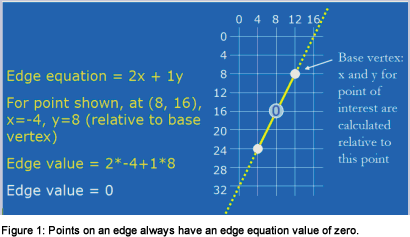Points on one side of the edge will have positive values, as in Figure 2 for the point at (12, 16), which has a value of 8.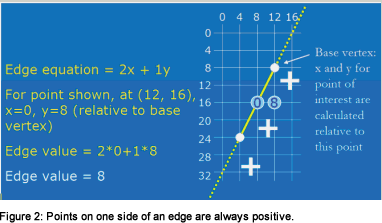Points on the other side of the edge will have negative values, as in Figure 3 for the point at (4, 12), which has a value of -12.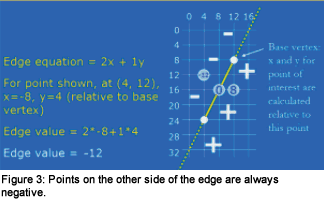Simple though it is, the edge equation is the basis upon which the Larrabee rasterizer is built. By applying the three edge equations at once, it is possible to determine which points are inside a triangle and which are not. Figure 4 shows an example of how this works; the pixels shown in green are considered to be inside the triangle formed by the edges, because their centers are inside all three edges. As you can see, the edge equation is negative on the side of each edge that's inside the triangle; in fact, it gets more negative the farther you get from the edge on the inside, and more positive the farther you get from the edge on the outside.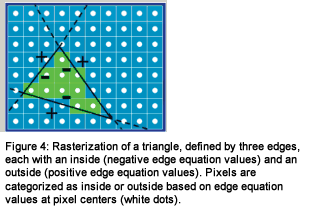Vectorization is an essential part of Larrabee performance - capable of producing a speedup of an order of magnitude or more - so the knotty question is how we can perform the evaluation in Figures 1-4 using vector processing. More accurately, the question is how we can efficiently perform the evaluation using vector processing; obviously, we could use vector instructions to evaluate every pixel on the screen for every triangle, but that would involve a lot of wasted work. What's needed is some way of using vector instructions to quickly narrow in on the work that's really needed.

We considered a lot of approaches. Let's take a look at a couple so you can get a sense of what a different tack we had to take in order to vectorize a task that's not an obvious candidate for parallelization - and to leverage the unique strengths of CPUs.

### The Pixomatic 1 Rasterization Approach

Pixomatic version 1 used a rasterization approach often used by scalar software rasterizers, decomposing triangles into 1 or 2 trapezoids, then stepping down the two edges simultaneously, on pixel centers, emitting the spans of pixels covered on each scan line, as in Figure 5.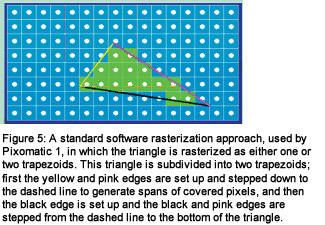This approach was efficient for scalar code, but it just doesn't lend itself to vectorization. There were several other reasons this approach didn't suit Larrabee well (for example, it emits pixel-high spans; but for vectorized shading, you want 4×4 blocks, both to generate 2D texture gradients and because a square aspect ratio results in the highest utilization of the vector units). But the most important reason was that I just could never come up with a way to get good results out of vectorizing edge stepping.

### Sweep Rasterization

Another approach, often used by hardware, is sweep rasterization. An example of this is in Figure 6. Starting at a top vertex, a vector stamp of 4×4 pixels is swept left, then right, then down, and the process is repeated until the whole triangle has been swept. The edge equation is evaluated directly at each of the 16 pixels for each 4×4 block that's swept over.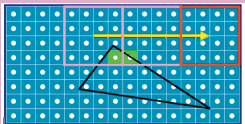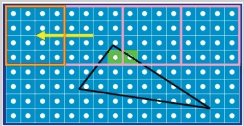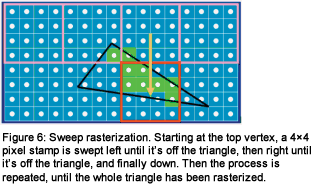Sweep rasterization is more vectorizable than the Pixomatic 1 approach because evaluating the pixel stamp is well-suited to vectorization; but on the other hand, it requires lots of badly predicted branching, as well as a significant amount of work to decide where to descend. It also fails to take advantage of the ability of CPUs to make smart, flexible decisions, which was our best bet for being competitive with hardware rasterization. So we decided sweep rasterization wasn't the right answer.

### A High-level View of Larrabee Rasterization

Larrabee takes a substantially different approach, one better suited to vectorization. In the Larrabee approach, we evaluate 16 blocks of pixels at a time to figure out which blocks are even touched by the triangle, then descended into each block that's at least partially covered, evaluating 16 smaller blocks within it, continuing to descend recursively until we had identified all the pixels inside the triangle. Here's an example of how that might work for our sample triangle.

As I'll discuss shortly, the Larrabee renderer uses a chunking architecture. In a chunking architecture, the largest rasterization target at any one time is a portion of the render target called a "tile"; for this example, let's assume the tile is 64×64 pixels, as in Figure 7.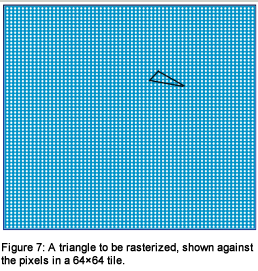First, we test which of the 16×16 blocks (16 of them - we check 16 things at a time whenever possible in order to leverage the 16-wide vector units) that make up the tile are touched by the triangle, as in Figure 8.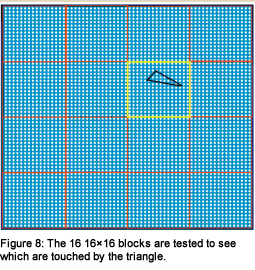We find that only one 16×16 block is touched - the block shown in yellow. So we descend into that block to determine exactly what is touched by the triangle, subdividing it into 16 4×4 blocks (once again, we check 16 things at a time to be vector-friendly), and evaluate which of those are touched by the triangle, as in Figure 9.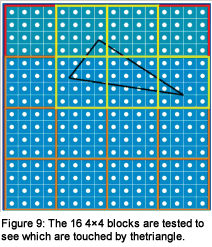We find that five of the 4×4s are touched, so we process each of them separately, descending to the pixel level to generate masks for the covered pixels. The pixel rasterization for the first block is in Figure 10.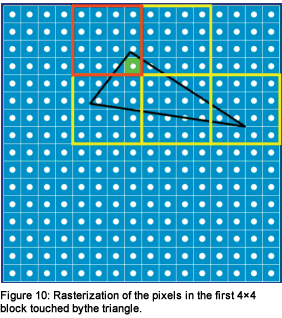Figure 11 shows the final result.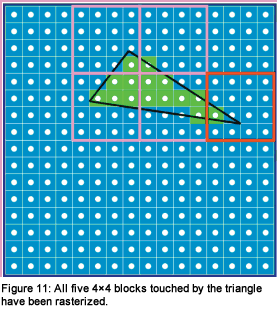As you can see, the Larrabee approach processes 4×4 blocks, like the sweep approach. But unlike the sweep approach, it doesn't have to make many decisions in order to figure out which blocks are touched by the triangle, thanks to the single 16-wide test performed before each descent. Consequently, this rasterization approach typically does somewhat less work than the sweep approach to determine which 4×4 blocks to evaluate. The real win, however, is that it takes advantage of CPU smarts by not-rasterizing whenever possible. I'll have to walk through the Larrabee rasterization approach in order to explain what that means, but as an introduction, let me tell you another optimization story.

Many years ago, I got a call from a guy I had once worked for. He wanted me to do some consulting work to help speed up his new company's software. I asked him what kind of software it was, and he told me it was image processing software, and that the problem lay in the convolution filter, running on a Sparc processor. I told him I didn't know anything about either convolution filters or Sparcs, so I didn't think I could be of much help. But he was persistent, so I finally agreed to take a shot at it.

He put me in touch with the engineer who was working on the software, who immediately informed me that the problem was that the convolution filter involved a great many integer multiplies, which the Sparc did very slowly because, at the time, it didn't have a hardware integer multiply instruction. Instead, it had a partial product instruction, which had to be executed for each significant bit in the multiplier. In compiled code, this was implemented by calling a library routine that looped through the multiplier bits, and that routine was where all the time was going.

I suggested unrolling that loop into a series of partial product instructions, and jumping into the unrolled loop at the right point to do as many partial products as there were significant bits, thereby eliminating all the loop overhead. However, there was still the question of whether to make the pixel value or the convolution kernel value the multiplier. The smaller the multiplier, the fewer partial products would be needed, so we wanted to pick whichever of the two was smaller on average.

When I asked which was smaller, though, the engineer said there was no difference. When I persisted, he said they were random. When I said that I doubted they were random (since randomness is actually hard to come by), he grumbled. I don't know why he was reluctant to get me that information - I guess he thought it was a waste of time - but he finally agreed to gather the data and call me back.

He didn't call me back that day, though. And he didn't call me back the next day. When he hadn't called me back the third day, I figured I might as well get it over with and called him. He answered the phone and, when I identified myself, he said, "Oh, Hi. I'm just standing here with my managers, watching. We're all really happy."

When I asked what exactly he was happy about, he replied, "Well, when I looked at the data, it turned out 90% of the values in the convolution kernel were zero, so I just put an if-not-zero around the multiply, and now the whole program runs three times faster!" Not-rasterizing is a lot like that, as we'll see shortly.

### Tile Assignment

As noted earlier, Larrabee uses "chunked" (also known as "binned" or "tiled") rendering, where the target is divided into multiple rectangles or tiles. The rendering commands are sorted according to the tiles they touch and stored in the corresponding bins, then the contents of each bin are rendered separately to the corresponding tile. It's a bit complex, but it considerably improves cache coherence and parallelization.

For chunking, rasterization consists of two steps: The first identifies which tiles a triangle touches, and the second rasterizes the triangle within each tile. So it's a two-stage process and I'm going to discuss the two stages separately.

Figure 12 shows an example of a triangle to be drawn to a tiled render target. The light blue area is a 256×256 render target, subdivided into four 128×128 tiles.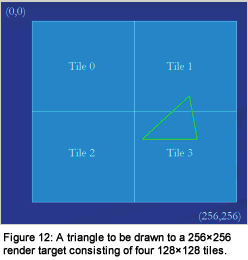With Larrabee's chunking architecture, the first step in rasterizing the triangle in Figure 12 is to determine which tiles the triangle touches and put the triangle in the bins for those tiles (tiles 1 and 3). Once all triangles have been binned, the second step, intra-tile rasterization, is to rasterize the triangle to tile 1 when the tile 1 bin is rendered, and to rasterize the triangle to tile 3 when the tile 3 bin is rendered.

Assignment of triangles to tiles can easily be performed for relatively small triangles - say, up to a tile in size, which covers 90% of all triangles - by doing bounding box tests. For example, it would be easy with bounding box tests to find out what two tiles the triangle in Figure 12 is in. Larger triangles are currently assigned to tiles by simply walking the bounding box and testing each tile against the triangle; that doesn't sound very efficient, but tile-assignment time is generally an insignificant part of total rendering time for larger triangles because there's usually a lot of shading work and the like to do for those triangles. However, if large-triangle tile-assignment time does turn out to be significant, we could use a sweep approach, as discussed earlier, or a variation of the hierarchical approach used for intra-tile rasterization, which I'll discuss next. This is a good example of how a CPU makes it easy to use two completely different approaches in order to do the 90% case well and the 10% case adequately (or well, but in a different way), rather than having to have one size fit all.

Large-triangle assignment to tiles is performed with scalar code for simplicity and because it's not a significant performance factor. Let's look at how that scalar process works because it will help us understand vectorized intra-tile rasterization later. I'll use a small triangle for the example to make the figures legible, but as previously noted, such a small triangle normally would be assigned to its tile or tiles using bounding box tests.

Once we've set up the equation for an edge (by calculating B and C, as discussed when we looked at Figure 1), the first thing we do is calculate its value at the trivial reject corner of each tile. The trivial reject corner is the corner at which an edge's equation is most negative within a tile; the selection of the trivial reject corner for a given edge is based on its slope, as we'll see shortly. We set things up so that negative means "inside" in order to allow us to generate masks directly from the sign bit. So you can think of the trivial reject corner as the point in the tile that's most inside the edge. If this point isn't inside the edge, no point in the tile can be inside the edge, and therefore, the whole triangle can be ignored for that tile.

Figure 13 shows the trivial reject test in action. Tile 0 is trivially rejected for the black edge and can be ignored because its trivial reject corner is positive, and therefore, the whole tile must be positive and must lie outside the triangle. Meanwhile, the other three tiles must be investigated further. You can see here how the trivial reject corner is the corner of each tile most inside the black edge; that is, the point with the most negative value in the tile.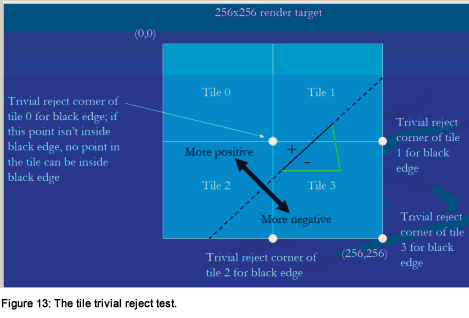Note that determining which corner is the trivial reject corner will vary from edge to edge depending on slope. For example, it would be the lower left corner of each tile for the edge shown in red in Figure 14 because that's the corner that's most inside that edge.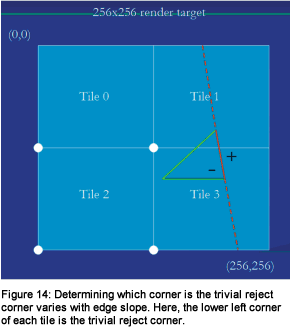If you understand what we've just discussed, you're ninety percent of the way to understanding the whole Larrabee rasterizer. The trivial reject test is actually very straightforward once you understand it - it's just a matter of evaluating the sign of a simple equation at the right point - but it can take a little while to get it, so you may find it useful to re-read the previous section if you're at all uncertain or confused.

So that's the tile trivial reject test. The other tile test is the trivial accept test. For this, we take the value at the trivial reject corner (the corner we just discussed) and add the amount that the edge equation changes for a step all the way to the diagonally opposite tile corner, the tile trivial accept corner. This is the point in the tile where the edge equation is most positive. You can think of this as the point in the tile that's most outside the edge. If the trivial accept corner for an edge is negative, that whole tile is trivially accepted for that edge and there's no need to consider that edge when rasterizing within the tile.

Figure 15 shows the trivial accept test in action. Because the trivial accept corner is the corner at which the edge's equation is most positive, if this point is negative - and therefore inside the edge - all points in the tile must be inside the edge. Thus, tiles 0 and 1 are not trivially accepted for the black edge, because the equation for the black edge is positive at their trivial accept corners, but tiles 2 and 3 are trivially accepted, so rasterization of this triangle in tiles 2 and 3 can ignore the black edge entirely, saving a good bit of work.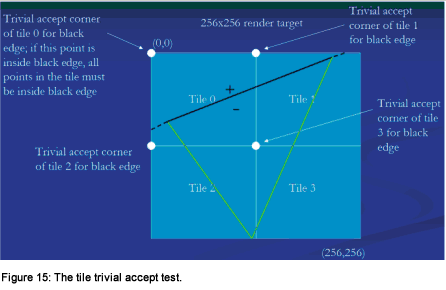There's an important asymmetry here. When we looked at trivial reject, we saw that it applies to the whole triangle in the tile being drawn to, in the sense that trivially rejecting a tile against any edge means the triangle doesn't touch that tile, so the triangle can be ignored in that tile. However, trivial accept applies only to the edge being checked; that is, trivially accepting a tile against an edge only means that the specific edge doesn't have to be checked when rasterizing the triangle to that tile because the whole tile is inside that edge; it has no direct implication for the whole triangle. The tile may be trivially accepted against one edge, but not against the others. In fact, it may be trivially rejected against one or both of the other edges, in which case, the triangle won't be drawn to the tile at all. This is illustrated in Figure 16, where tile 3 is trivially accepted against the black edge, so the black edge wouldn't need to be considered in rasterizing the triangle to that tile, but tile 3 is trivially rejected against the red edge, and that means that the triangle doesn't have to be rasterized to tile 3 at all.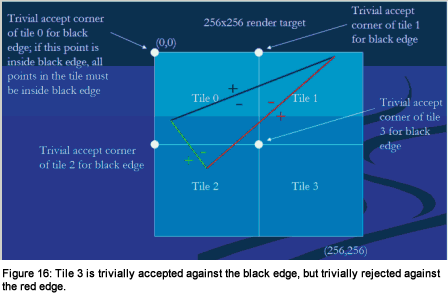In Figure 17, however, tile 3 is trivially accepted by all three edges, and here we come to a key point.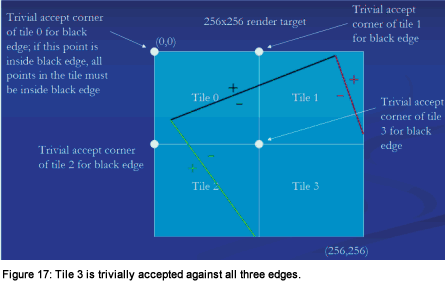If all three edges are negative at their respective trivial accept corners, then the whole tile is inside the triangle, and no further rasterization tests are needed - and this is what I meant earlier when I said the rasterizer takes advantage of CPU smarts by not-rasterizing whenever possible. The tile-assignment code can just store a draw-whole-tile command in the bin. Then the bin rendering code can simply do the equivalent of two nested loops around the shaders, resulting in a fullscreen triangle rasterization speed of approximately infinity - one of my favorite performance numbers!

By the way, this whole process should be familiar to 3D programmers because testing of bounding boxes against planes (for example, for frustum culling) is normally done in exactly the same way - although in three dimensions instead of two - with the same use of signs to indicate inside and outside for trivial accept and reject. Also, structures such as octrees employ a 3D version of the hierarchical recursion used by the Larrabee rasterizer.

That completes our overview of how rasterization of large triangles for tile assignment works. As I said, this is done as a scalar evaluation in the Larrabee pipeline, so the trivial accept and reject tests for each tile are performed separately. Intra-tile rasterization, to which we turn next, is much the same, but vectorized. And it is this vectorization that will give us insight into applying the Larrabee New Instructions to semi-parallel tasks.

### Intra-tile Rasterization: 16×16 Blocks

Intra-tile rasterization starts at the level of a whole tile. Tile size varies, depending on factors such as pixel size, but let's assume that we're working with a 64×64 tile. Given that starting size, we calculate the edge equation values at the 16 trivial reject and trivial accept corners of the 16×16 blocks that make up the tile, just as we did at the tile level - but now we do these calculations 16 at a time. Let's start with the trivial reject test.

First, we calculate which corner of the tile is the trivial reject corner, calculate the value of the edge equation at that point, and set up a table containing the 16 steps of the edge equation from the value at the tile trivial reject corner to the trivial reject corners of the 16×16 blocks that make up the tile. The signs of the 16 values that result tell us which of the blocks are entirely outside the edge, and can therefore be ignored, and which are at least partially accepted, and therefore have to be evaluated further.

In Figure 18, for example, we calculate the trivial reject values for the black edge by stepping from the value we calculated earlier at the trivial reject corner of the tile, and eliminate five of the 16×16 blocks that make up the tile. The trivial reject corner for the tile is shown in red, and the 16 trivial reject corners for the blocks are shown in white. The gray blocks are those that are rejected against the black edge. You can see that their trivial reject corners all have positive edge equation values. The other 11 blocks have negative values at their trivial reject corners, so they're at least partially inside the black edge.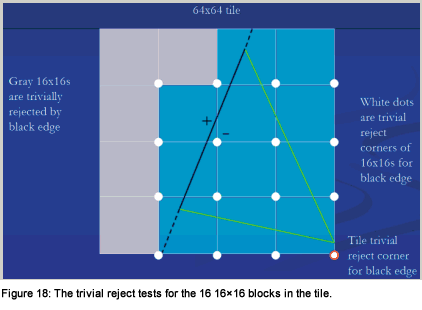To make this process clearer, in Figure 19, the arrows represent the 16 steps that are added to the black edge's tile trivial reject value. Each of these steps is just an add, and we can do 16 adds with a single vector instruction, so it takes only one vector instruction to generate the 16 trivial reject values, and one more vector instruction - a compare - to test them.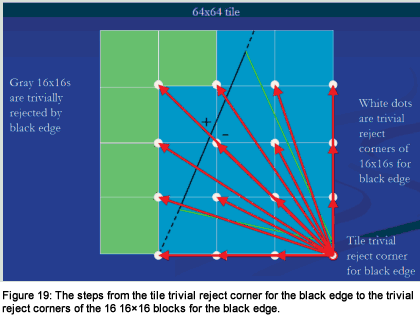All this comes down to just setting up the right values, then doing one vector add and one vector compare. Remember that the edge equation is of the form Bx + Cy; therefore, to step a distance across the tile, we just set x and y to the horizontal and vertical components of that distance, evaluate the equation, and add that to the starting value. So all we're doing in Figure 19 is adding the 16 values that step the edge equation to the 16 trivial reject corners. For example, to get the edge equation value at the trivial reject corner of the upper- left block, we'd start with the value at the tile trivial reject corner, and add the amount that the edge equation changes for a step of -48 pixels in x and -48 pixels in y, as shown by the yellow arrow in Figure 20. To get the edge equation value at the trivial reject corner of the lower-left block, we'd instead add the amount that the edge equation changes for a step of -48 pixels in x only, as shown by the purple arrow. And that's really all there is to the Larrabee rasterizer - it's just a matter of stepping the edge equation values around the tile so as to determine what blocks and pixels are inside and outside the edges.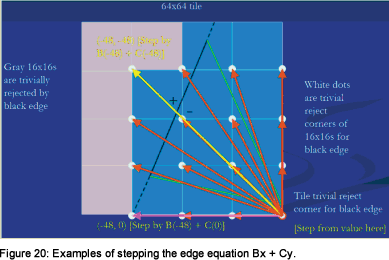Once again, we'll do trivial accept tests as well as trivial reject tests. In Figure 21, we've calculated the trivial accept values and determined that 6 of the 16×16 blocks are trivially accepted for the black edge, and 10 of them are not trivially accepted. We know this because the values of the equation of the black edge at the trivial accept corners of the 6 pink blocks are negative, so those blocks are entirely inside the edge; while the values at the trivial accept corners of the other 10 blocks are positive, so those blocks are not entirely inside the black edge. The trivial accept values for the blocks can be calculated by stepping in any of several different ways: from the tile trivial accept corner, from the tile trivial reject corner, or from the 16 trivial reject values for the blocks. Regardless, it again takes only one vector instruction to step and one vector instruction to test the results. Combined with the results of the trivial reject test, we also know that 5 blocks are partially accepted.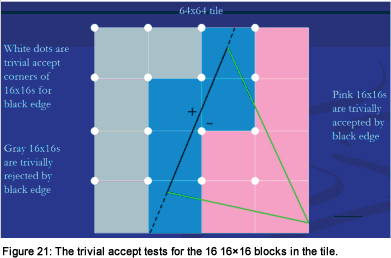The 16 trivial reject and trivial accept values can be calculated with a total of just two vector adds per edge, using tables generated at triangle set-up time,. They can be tested with two vector compares, which generate mask registers describing which blocks are trivially rejected and which are trivially accepted. We do this for the three edges, ANDing the results to create masks for the triangle, do some bit manipulation on the masks so they describe trivial and partial accept, and bit-scan through the results to find the trivially and partially accepted blocks. Each 16×16 that's trivially accepted against all three edges becomes one bin command; again, no further rasterization is needed for pixels in trivially accepted blocks.

This is not obvious stuff, so let's take a moment to visualize the process. First, let's just look at one edge and trivial accept.

For a given edge, say edge number 1, we take the edge equation value at the tile trivial accept corner, broadcast it out to a vector, and vector add it to the precalculated values of the 16 steps to the trivial accept corners of the 16×16 blocks. This gives us the edge 1 values at the trivial accept corners of the 16 blocks, as in Figure 22. (The values shown are illustrative, and are not taken from a real triangle.)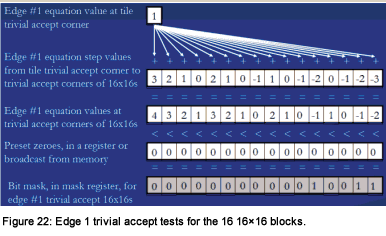The step values shown on the second line in Figure 22 are computed when the triangle is set up, using a vector multiply and a vector multiply- add. At the top level of the rasterizer - testing the 16×16 blocks that make up the tile, as in Figure 22 - those set-up instructions are just direct additional rasterization costs because the top level only gets executed once per triangle, so it would be accurate to add 6 instructions to the cost of the 16×16 code we'll look at shortly in Listings One and Two. However, as the hierarchy is descended, the tables for the lower levels (16×16-to-4×4 and 4×4-to-mask) get reused multiple times. For example, when descending from 16×16 to 4×4 blocks, the same table is used for all partial 16×16 blocks in the tile. Likewise, there is only one table for generating masks for partial 4×4 blocks, so the additional cost per iteration in Listing 3 due to table set-up would be 2 instructions divided by the number of partial 4×4 blocks in the tile. This is generally much less than 1 instruction per 4×4 block per edge, although it gets higher the smaller the triangle is.

Then we compare the results to 0; as in Figure 22, this generates a mask that contains 1-bit where a block trivial accept corner is less than zero - that is, wherever a block trivial accept corner is inside the edge, meaning the whole block is inside the edge.

We do this for each of the three edges, ANDing the masks together to produce a composite mask, as in Figure 23. (The ANDing happens automatically as part of the mask updating, as we'll see later when we look at the code.) This composite mask has 1-bit only where a block trivially accepts against all three edges, which is to say, for blocks that are fully trivially accepted and require no further rasterization.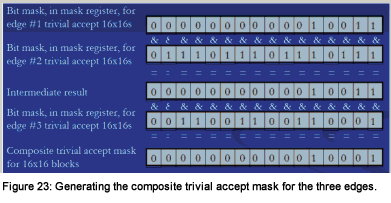Now we can bit-scan through the composite mask, picking out the 16×16 blocks that are trivially accepted and storing a draw-block command for each of them in the rasterizer output queue. The first bit-scan would find that block 0 is trivially accepted, the second bitscan would find that block 4 is trivially accepted, and the third bit-scan would find that there were no more trivially accepted blocks, as in Figure 24.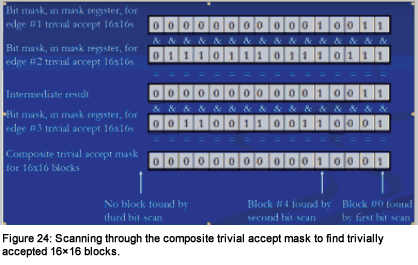This sort of parallel-to-serial conversion - identifying and processing relevant elements of a vector result efficiently - is key to getting good performance out of semi-vectorizable code. This is exactly why the bsfi instruction was added as part of the Larrabee New Instructions. Bsfi is based on the existing bit-scan instruction, but is enhanced to allow starting the scan at any bit, rather than only at bit 0.

We're finally ready to look at some real code. Listing One shows just the trivial accept code for the 16×16 blocks in a 64×64 tile; this will suffice to illustrate what we've discussed, and anything more would just complicate the explanation. Let's map these instructions to the steps we just described.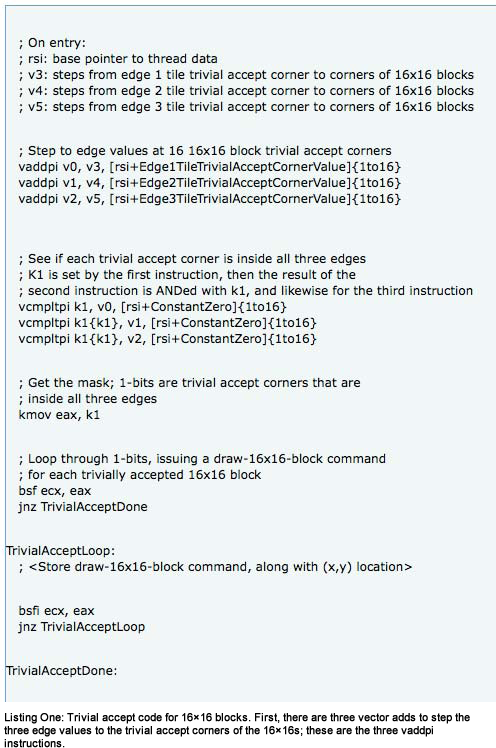Second, there are three vector compares to test the signs of the results to see if the blocks are trivially accepted against the edges; these are the three vcmpltpi instructions. The composite mask is accumulated in mask register k1.

Next, the kmov copies the mask of trivially accepted blocks into register eax; and finally, there is a loop to bit-scan eax to pick out the trivially accepted 16×16s one by one so they can be processed as blocks without further rasterization.

It takes only six vector instructions, one mask move, and two scalar instructions to set up, plus two scalar instructions per trivially accepted block, to find all the trivially accepted 16×16s in a tile. And, in truth, it doesn't even take that many instructions. What's shown in Listing 1 is a version of the trivial accept code that uses normal vector instructions, but there's actually a special instruction specifically designed to speed up rasterization - vaddsetspi - that both adds and sets the mask to the sign. I haven't mentioned it because I thought the discussion would be clearer and more broadly relevant if I used the standard vector instructions, but as you can see in Listing Two, if we use vaddsetspi, we need only three vector instructions to find all the trivially accepted 16×16 blocks.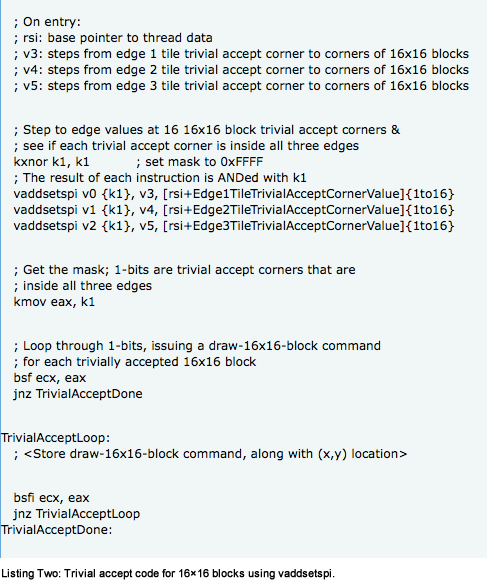There may also be fewer active edges due to trivial accepts at the tile level (remember, edges that are trivially accepted for a tile can be - and are - ignored when rasterizing within the tile), and that would result in still fewer instructions. This is another case where software can boost performance by adapting to the data.

### Descending the Rasterization Hierarchy

We're now done rasterizing trivially accepted 16×16 blocks, but we still have to handle partially covered 16×16 blocks, and by this point, it should be obvious how that works. We descend into each partial 16×16 to evaluate the 4×4 blocks it contains, just as we descended into the 64×64 tile to evaluate the 16×16s it contained. Again, we put trivially accepted 4×4s directly into the bin. Partially accepted 4×4s, however, need to be processed into pixel masks. This is done with a vector add of a precalculated, position-independent table for each edge that steps from the 4×4 trivial reject corner to the center of the pixel itself. The sign bits can then be ANDed together to form the pixel mask.

Figure 25 illustrates the calculation of the pixel mask. From the trivial reject corner of the 4×4, shown in red, a single add yields the equation value for the black edge at the 16 pixel centers. The red arrows show how the 16 values in the precalculated, position-independent step table for each edge are added to the trivial reject corner of the 4×4 to evaluate the edge equation at the 16 pixel centers. The pixels shown in blue have negative values and are inside the edge. Figure 25 shows the actual mask that is generated, with a 1-bit for each pixel inside the edge, and a 0-bit for each pixel that's outside.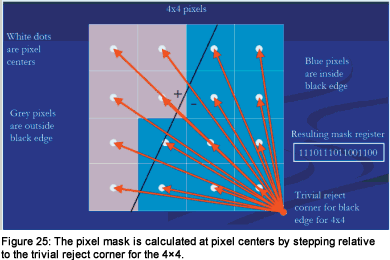Figure 25 is a good demonstration of how vector efficiency can fall off with partially vectorizable tasks, and why Larrabee uses 16-wide vectors rather than something larger. We will often do all the work needed to generate the pixel mask for a 4×4, only to find that many or most of the pixels are uncovered, so much of the work has been wasted. And the wider the vector, the lower the ratio of covered to uncovered becomes, on average, and the more work is wasted.

Listing Three shows code for calculating the pixel masks for all the partially covered 4×4 blocks in a partially covered 16×16 block. Note that this is the only case in which per-pixel work is performed; all solidly covered-block cases are handled without generating a pixel mask.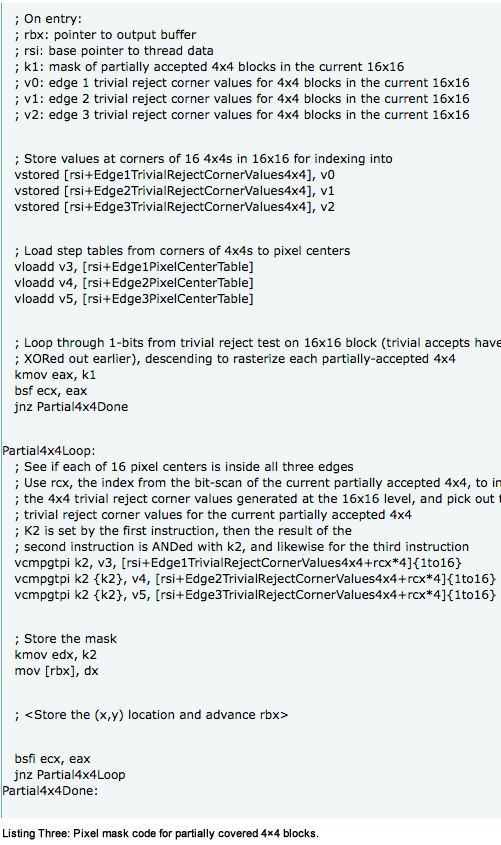Listing Three first stores the trivial reject corner values for the 16 4×4 blocks for the three edges. These are the values we'll step relative to in order to generate the final pixel masks for each partial 4×4 and that were generated earlier by the 16×16 code that ran just before this code. This is done with the three vstored instructions.

Next, the step tables for the three edges are loaded with the three vloadd instructions. (Actually, these will probably just be preloaded into registers when the triangle is set up and remain there throughout the rasterization of the triangle, but loading them here makes it clearer what's going on.)

Once that set-up is complete, the code scans through the partial accept mask, which was also generated earlier by the 16×16 code. First, the mask is copied to eax with the kmov instruction, and then bsfi is used to find each partially accepted 4×4 block in turn.

For each partially covered 4×4 found, the code does three vector compares to evaluate the three edge equations at the 16 pixel centers. Note that each vcmpgtpi uses the index of the current 4×4 to retrieve the trivial reject corner value for that 4×4 from the vector generated at the 16×16 level. While we could directly add and then test the signs of the edge equations, as I described earlier, it's more efficient to instead rearrange the calculations by flipping the signs of the step tables so that the testing can be done with a single compare per edge. Put another way, instead of adding two values and seeing if the result is less than zero:

m + n < 0

it's equivalent and more efficient to compare one value directly to the negation of the other value:

m < -n

(In case you're wondering, we couldn't use this trick at the 16×16 and 64×64 levels of the hierarchy because in those cases, in addition to getting the result of the test, we also need to keep the result of the add, so we can pass it down the hierarchy as the new corner value.)

The result of the compares is the final composite pixel mask for that 4×4, which can be stored in the rasterization output queue. And that's it. Once things are set up, the cost to rasterize each partial 4×4 is three vector instructions, a mask instruction, and a handful of scalar instructions. Once again, if the whole tile was trivially accepted against one or two edges, then proportionately fewer vector instructions would be required.

One nice feature of the Larrabee rasterization approach is that MSAA ("multisample antialiasing") falls out for one extra vector compare per 16 samples per edge because it's just a different step from the trivial reject corner. (That's for partially accepted 4×4 blocks; for all trivially accepted blocks, there is no incremental cost for rasterization of MSAA.) In Figure 26, each pixel is divided into four samples, and the step shown is to one of the four samples rather than to the center of each pixel. This takes one compare, so a total of four compares would be required to generate the full MSAA sample mask for 16 pixels.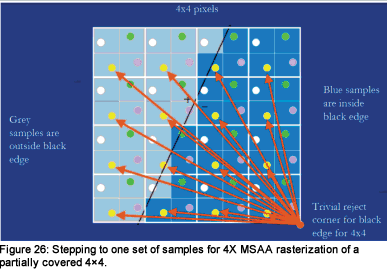### Pulling It All Together

Now that we've stepped all the way down through the rasterization hierarchy, let's go back and look again at the rasterization descent overview we started with, this time with a detailed understanding what's going on.

Figure 27 shows a triangle and a 64×64 tile to which the triangle is to be drawn, with the tile subdivided into 16×16 blocks; Figure 27 is a repeat of Figure 8, but this time I've added dashed extensions of the edges to the border of the tile, so we can see what blocks and pixels are on what sides of the edges.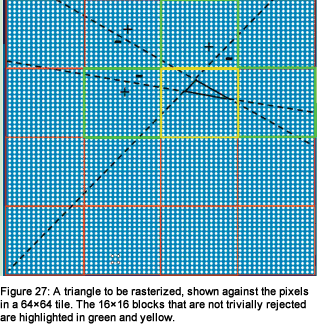To rasterize the triangle in Figure 27, we first calculate the values of the triangle's three edge equations at the tile's trivial accept and trivial reject corners and find that the tile is neither trivially rejected nor trivially accepted by any edge. (Again, this would actually only be done for a large triangle; we would use bounding box tests for such a small triangle.) We set up the various step tables we'll use, and then we step the edge equations to their respective trivial accept and trivial reject corners of the 16 blocks, each 16×16 in size, that make up the tile, and make a mask containing the signs of the results.

We then bit-scan through the resulting mask, find that 12 of the 16 blocks are trivially rejected, and descend into each of the remaining 4 blocks in turn. In three of the blocks, we'll ultimately find that there's nothing to draw, so for the purposes of this discussion, we'll ignore those and look at the more interesting case of what happens when we descend into the block that the triangle lies inside - the block outlined in yellow. (Note that if the triangle were large enough to fully cover a 16×16 block, that block would be trivially accepted and no further descent into that block would be required.)

Before we look at what happens when we descend into the 16×16 block containing the triangle, there's one more thing in Figure 27 that we should examine. You may have noticed that in the earlier version of this figure, Figure 8, only the one block in yellow was found, not the three green blocks. Why did the bit-scan find 4 blocks this time, when the triangle is entirely contained in one block? The reason is that the Larrabee rasterization approach, as discussed in this article, can only eliminate blocks by trivially rejecting them. If you look closely, you will see that none of the three green blocks is trivially rejected by any edge. This is an inefficiency of this rasterization method, although there are techniques, which are beyond the scope of this article, that remove much of the waste.

Descending the rasterization hierarchy, we take the 16×16 block containing the triangle, subdivide it into 16 4×4 blocks, and evaluate which of those are touched by the triangle by stepping to evaluate the edge equation at each of their trivial accept and trivial reject corners for each edge, as in Figure 28. We find that 10 of the blocks are trivially rejected, and that none of the 6 remaining blocks are trivially accepted against all three edges.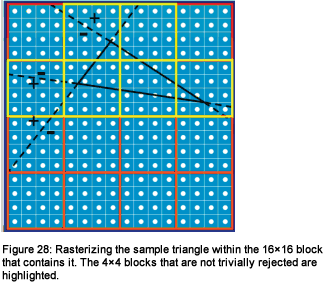We've finally reached the bottom of the rasterization hierarchy, so we can bit-scan through the partial-accept mask generated for the 16×16, to find the partially accepted 4×4 blocks, and generate the 4×4 pixel mask for each of the blocks in turn, as in Figure 29.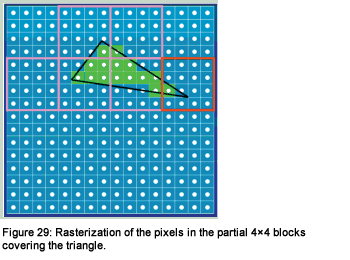Here, we see once again that the reliance on trivial reject to eliminate blocks has caused a false positive on a block that actually doesn't touch the triangle (the left-most block). It's possible to do bounding box tests to eliminate such blocks, but it's not clear whether that's more efficient than just testing for empty masks - that is, masks with no pixels enabled - and skipping those blocks.

After completing this 16×16 block, we pop back up to rasterize the other 16×16 blocks that weren't trivially rejected (which in this case, turned out not to contain any of the triangle). And that's really all there is to it!

### Notes on Rasterization

Now that we understand the basic rasterization algorithm, let's take a quick look at some interesting implementation refinements.

In software, we don't have the luxury of custom data and ALU sizes, but we do have the luxury of adapting to input data, and this adaptive rasterization helps boost our efficiency. For example, edge evaluations have to be done with 48 bits in the worst case. For those cases, being software, we have to use 64 bit because there is no 48-bit integer support in Larrabee. However, we don't have to do that at all for the 90+% of all triangles that fit in a 128×128 bounding box because, in those cases, 32 bits is enough.

When we do have to do 64-bit edge evaluation, we only have to use it for tile assignment. As it turns out, within tiles up to 128×128 in size (and 128×128 is our largest tile size), any edge that the tile is not trivially accepted or rejected against can always be rasterized using 32 bits.

We can also detect triangles that fit in a 16×16 bounding box and process them with one less descent level, less set-up, and no trivial accept test (because there will rarely be trivially accepted 4×4s in such small triangles). Finally, triangles that fit in very small bounding boxes can be done simply by directly calculating the masks for the 16 or 32 pixels directly, with little set-up and minimal processing.

In fact, for small triangles we could even take the z value of the closest vertex and compare it to the z buffer for the triangle's bounding box, and possibly z-reject the triangle before we even rasterize it!

There are other optimization possibilities I won't get into because there's just not space in this article, and of course, there's no telling how well they'll work until we try them. But one nice thing about software is that it's easy to run the experiments to check them out.

### Final Thoughts

And with that, we conclude our lightning tour of the Larrabee rasterization approach, and our examination of how vector programming can be applied to a semi-parallel task. As I mentioned earlier, software rasterization will never match dedicated hardware peak performance and power efficiency fora given area of silicon, but so far, it's proven to be efficient enough. It also has a significant advantage, in that because it uses general-purpose cores, the same resources that are used for rasterization can be used for other purposes at other times, and vice versa.

As Tom Forsyth puts it, because the whole chip is programmable, we can effectively bring more square millimeters to bear on any specific task as needed - up to and including the whole chip. In other words, the pipeline can dynamically reconfigure its processing resources as the rendering workload changes. If we get a heavy rasterization load, we can have all the cores working on it.

It wouldn't be the most efficient rasterizer per square millimeter, but it would be one heck of a lot of square millimeters of rasterizer, all doing what was most important at that moment; in contrast to a traditional graphics chip with a hardware rasterizer, where most of the circuitry would be idle when there was a heavy rasterization load. A little while later, when the load switches to shading, the whole Larrabee chip can become a shader if necessary. Software simply brings a whole different set of strengths and weaknesses to the table.

There's a lot to learn and rethink with Larrabee, and a lot of potential to be exploited. Only time will tell how well it all works out - but meanwhile, it certainly is an interesting time to be a performance programmer!

Further information about Larrabee is available at www.intel.com/software/graphics.

Michael Abrash is a programmer at RAD Game Tools working on the Larrabee project, a video game programming veteran, and the author of numerous books and articles on graphics programming and performance optimization.

[This article was originally published in association with Gamasutra's sister publication Dr. Dobb's, which delivers in-depth coverage of the art and business of software development from a cross-platform, language-independent point of view. Dr. Dobb's Report (print), Digest (digital) and Online all contain articles written exclusively for professional software development leaders and architects, and provides tools and techniques using relevant, real-world solutions.]

### Latest Jobs

#### Infinity Ward

Woodland Hills, California
11.3.21
Sr. Multiplayer Design Scripter/Programmer

#### Disbelief

Cambridge, Massachusetts
11.3.21
Jr. Programmer

#### 4A Games

Malta
11.3.21
Lead Game Systems Designer New IP

#### XSEED

Torrance, California
11.3.21
More Jobs